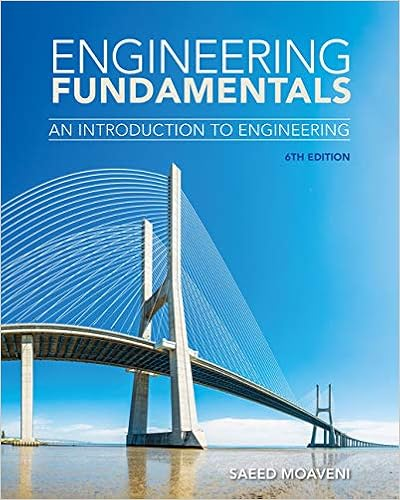# 10 silicon diodes are used in a two diode full wave

• Homework Help
• 3
• 98% (85) 83 out of 85 people found this document helpful

This preview shows page 2 - 3 out of 3 pages.

##### We have textbook solutions for you!
The document you are viewing contains questions related to this textbook.The document you are viewing contains questions related to this textbook.
Chapter 3 / Exercise 3.37
Engineering Fundamentals: An Introduction to Engineering
MoaveniExpert Verified
10. Silicon diodes are used in a two-diode full-wave rectifier circuit to supply a load with 12 volts D.C. Assuming ideal diodes and that the load resistance is 12 ohms, compute the secondarytransformer voltage, the load ripple voltage, and the efficiency of the rectifier. Show all work.
s,
.11. A half-wave rectifier using silicon diode has a secondary emf of 14.14 V (rms) with a resistance of 0.2 Ω. The diode has a forward resistance of 0.05 Ω and a threshold voltage of 0.7 V. If load resistance is 10 Ω, determine the following: to start with I need to find the Vm which is14.14*2= 28.8 ◦dc load current20-0.7/10.25= 0.6A◦dc load voltage 6V◦voltage regulation 28.28-27.58/27.58 *100= 2.5%◦circuit efficiency 8.78/14.14= 62% CORRECT ANSWER IS
##### We have textbook solutions for you!
The document you are viewing contains questions related to this textbook.The document you are viewing contains questions related to this textbook.
Chapter 3 / Exercise 3.37
Engineering Fundamentals: An Introduction to Engineering
MoaveniExpert Verified
•••